# Earth’s Non-Thermal Energy Storage Rate

The planetary temperature formula includes a term,, which represents the net rate at which energy in the planet’s “climate zone” is being stored as non-thermal energy (i.e., energy not reflected in the temperature or phase of matter).

How large is the rate of non-thermal-energy storage on Earth at present?

In what follows, I will estimate the rates at which energy is stored in the following non-thermal forms:

• Mechanical energy of atmospheric circulation
• Mechanical energy of ocean circulation
• Chemical energy of organic matter

Note that, conceptually, there is an “energy reservoir” associated with each type of energy. The net energy storage rate for that type of energy is the rate at which energy is converted to that type of energy (energy enters the reservoir), minus the rate at which that type of energy is converted to thermal energy (energy leaves the reservoir).

If an energy reservoir maintains the same amount of contained energy, with no net increase or decrease, then that type of energy would contribute nothing to the overall energy storage rate. The absolute size of the energy reservoir doesn’t matter to the storage rate,. Only the change in the amount of energy in the reservoir is relevant to this calculation.

For each quantity, I will express its magnitude in three different forms:

• Zettajoules/year (ZJ/yr) — global annual total change in energy (1 ZJ = 1021 J)
• Terawatts (TW) — global annual total change in energy divided by seconds in a year, or average global rate of energy change (1 TW = 1012 W)
• Watts/m2 (W/m2) — global annual total change in energy divided by seconds in a year and surface area of planet, or average rate of energy change per unit surface area

Each of these forms expresses the same information. The units are just different. The conversion factors are:

1 W/m² ⇔ 510 TW ⇔ 16.1 ZJ/yr

### How Large an Energy-Storage Rate is Significant?

My analysis CERES data indicates that over the period 2000-2021 a value of 1 W/m2 in the energy conservation equation has correspond to a temperature change of 0.300℃. Equivalently, a temperature change of 0.01℃ has corresponded to an energy flux of 0.033 W/m2.

So, if we apply a standard that we consider an energy-storage rate to be “significant” if if corresponds to at least 0.01℃, then we would be interested in whether the energy storage rate exceeds:

0.54 ZJ/yr
17 TW
0.033 W/m2

### Size of Overall Energy Storage Rate

The total energy-storage rate is given by: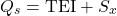where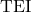is the top-of-atmosphere radiative energy imbalance and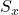is the rate of non-solar heating. So, we know that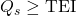.

The above numbers correspond roughly to the size of the measured TOA radiative energy imbalance, TEI, in recent years. Note that the overall energy storage rate,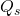, must be at least that large, since≥ TEI.

CERES data indicates that 12-month values ofhave ranged from about zero to 1.7 W/m2 over the period 2000-2021. So, 12-month values ofhave been at least this large.

So, non-thermal energy storage rates would need to be at least a few percent of this 1.7 W/m2 value in order to alter any calculations in a significant way.

### Mechanical Energy of Atmospheric Circulation

Earth’s atmosphere functions as a heat engine, driven by what is called the Lorenz energy cycle. In this cycle, temperature and moisture differences create convective potential energy, which is converted to kinetic energy of moving air. Energy enters the cycle via absorption of heat from sunlight, and leaves the cycle as heat through dissipation (i.e., friction).

There will be a net energy storage rate associated with atmospheric mechanical energy if the amount of energy tied up in atmospheric circulation is increasing over time.

Pan et al. (2017) estimate that the total potential plus kinetic energy of atmospheric circulation has increased with a linear trend rate of 1158.8 ±1985.3 J/m²/yr over the period 1979-2014 (see supplementary figure 10).

In our standard units, this equates to a net energy storage rate of:

(5.9  ± 10) × 10-4 ZJ/yr
(1.9  ± 3.2) × 10-2 TW
(3.7  ± 6.3) × 10-5 W/m2

This value has quite a bit of uncertainty. However, even taking that uncertainty into account, the rate of energy storage as atmospheric mechanical energy is quite small compared to the reference value of 0.033 W/m2 for a 0.01℃ temperature change.

That’s the conclusion for the long term trend. What about year-to-year variations in atmospheric mechanical energy?

The same source suggests that, between one year and the next, random fluctuations in atmospheric mechanical energy might vary over a range of up to about 3×10⁵ J/m2/yr. In our standard units, this equates to short term fluctuations in the energy storage rate up to about:

±0.15 ZJ/yr
±4.9 TW
±0.0095 W/m2

So, annual fluctuations in the rate of storage as atmospheric mechanical energy are much larger than the long term trend. Yet, the value is still smaller than the 0.033 W/m2 reference value.

### Mechanical Energy of Ocean Circulation

The ocean too has a circulation system that stores energy.

Various studies have reported the energy associated with this circulation has been increasing overall in recent decades (though some individual currents have weakened).

Hu et al. (2020) estimate that for the increase in the energy of ocean circulation the “mean trend over the post-1990 period is approximately (1.37 ± 1.10) × 1017 J/decade and is significantly different from zero at the 99% confidence level. This rate of change is equivalent to an increase of (15 ± 12)% /decade over the climatological mean of 9.05 × 1017 J.”

In our standard units, that equates to an energy storage rate of:

(1.4  ± 1.1) × 10-5 ZJ/yr
(4.3  ± 3.5) × 10-4 TW
(8.5  ± 6.8) × 10-7 W/m2

The rate of energy storage into mechanical energy of ocean circulation appears to be much smaller than the rate of energy storage into atmospheric mechanical energy. It seems unlikely to be important to consider storage of oceanic circulation mechanical energy `when considering the overall energy storage rate.

### Chemical Energy of Organic Matter

Photosynthesis converts solar energy into chemical energy within organic matter. That energy is later converted to heat when organic matter is decomposed.

If the total amount of organic matter on the planet was increasing, that would constitute a form of net energy storage.

Wikipedia estimates that photosynthesis currently produces about 123 GtC/yr (gigatonnes of carbon/year) while about 118.7 +1.1 = 119.8 GtC/yr of organic matter is returned to the atmosphere. (See this figure. Note that 1 Gt = 1 Pg.) This implies a net increase in organic carbon of about 3.2 GtC/yr.

Wikipedia also reports that photosynthesis capturing 130 TW of energy over a year equates to producing about 98 GtC of organic matter. Using that proportionality, 3.2 GtC/yr would equate to a net energy storage rate of 4.3 TW.

So, in our standard units, the energy storage rate associated with storing energy as chemical energy in organic matter is roughly:

4.3 TW
0.14 ZJ/yr
0.0084 W/m2

This is the most significant non-thermal energy storage mechanism we have examined (if we are considering sustained levels over multiple years). So, if we are going to take into account any non-thermal energy storage mechanism, it should be this one.

However, this value is still smaller than the reference value of 0.033 W/m2 for a 0.01℃ temperature change.

### Overall Non-Thermal-Energy Storage Rate

Summing the different non-thermal-energy storage rates we have considered, the long-term average ofseems likely to be around 0.008 W/m2. There may be year-to-year fluctuations around this value of ±0.0095 W/m2 (or possibly larger, given that we haven’t quantified fluctuations in the chemical-energy storage rate).

This value is much smaller than the overall energy storage rate,, which has gotten as large as 1.7 W/m2 in recent years. So, it appears that the energy stored in Earth’s “climate zone” is mostly being stored as thermal energy, i.e., energy associated with the temperature or phase of matter.

I am not aware of any other non-thermal-energy storage mechanism that is likely to store energy at a rate large enough to significantly affect calculations of Earth’s temperature.1The burning of fossil fuels could be considered release of energy that has been stored chemically; however, I prefer to account for that process via the non-solar heating term,.

### Appendix: Gravitational potential energy associated with atmospheric expansion

As the atmosphere warms, it expands, in accordance with the ideal gas law,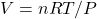. Since the atmosphere cannot expand horizontally, it expands vertically. This results in the center-of-mass of the atmosphere being somewhat higher, relative to the Earth’s surface. Changing the altitude of the atmosphere’s mass changes its gravitational potential energy.

One might wonder whether this needs to be addressed in our accounting of stored energy.

It turns out that the answer is, “no,” because it has already implicitly been accounted for.

Changes in gravitational potential energy are implicitly accounted for in the difference between the heat capacity for constant pressure,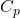, in comparison to the the heat capacity for constant volume,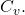is larger than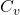because the former accounts for the work that needs to be done to lift the atmosphere against the force of gravity. So, when we calculate the energy needed to warm the atmosphere using, we are already taking gravitational potential energy into account in our accounting of “thermal” energy.